### IMO Shortlist 1988 problem 17

Kvaliteta:
Avg: 0,0
Težina:
Avg: 0,0
In the convex pentagon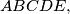$ABCDE,$ the sides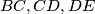$BC, CD, DE$ are equal. Moreover each diagonal of the pentagon is parallel to a side ($AC$ is parallel to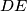$DE$,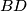$BD$ is parallel to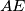$AE$ etc.). Prove that$ABCDE$ is a regular pentagon.
Izvor: Međunarodna matematička olimpijada, shortlist 1988